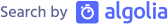CloseClose full mode# Object-Oriented-Programming in F#Edit on Github

# Introduction

How to write a class in F#? This chapter explains Object-oriented "capabilities" in F#. Warning, though, Object-Oriented feature is the second-class citizen in F#, meaning OOP model in F# is simpler than C#, for example there is no `protected` access level in F#... OOP in F# is just for interoperation with C# libraries.

# Class

## Simple Class

Let's create a simple `Point` class for comparison. Note that since F# uses immutable data types by default, the example is also immutable for simplicity sake.

`// Point.csusing System;namespace Example {    public class Point {        public int X { get; }        public int Y { get; }        public Point(int x, int y){            X = x;            Y = y;        }        /// <summary>        /// Distance of this point from origin (0,0)        /// </summary>        public double CalculateDistance() => Math.Sqrt(X*X + Y*Y);    }}`

In F#:

`// Point.fsmodule Exampletype Point(x: int, y: int) =    member my.X = x    member my.Y = y        /// Distance of this point from origin (0,0)    member my.CalculateDistance() = sqrt(double(me.X * me.X + me.Y * me.Y))`

Much shorter and more pleasant code, isn't it? 😆 Generally, writing a class in F# is shorter and terse due to its simpler syntaxes. To define a class, use keyword `type` following by a class name and constructor parameters. This line `type Point(x: int, y: int)` declares the class `Point` and the constructor in one line, neat, right?

Next 2 lines defines `X` and `Y` properties. Note that it can refer to the constructor's parameter right away! The word `my` is a custom identifier representing the object instance. You can use any name on this part, F# doesn't have keyword `this`. People often use the word `this` but, for me, I often use `me` or `my` as it's shorter. 😊

The last line is to define the method `CalculateDistance`, this one is very much like C#'s but shorter due to type omission, though you may notice that the method's body is longer. This is because `X` and `Y` are `int` but to use `sqrt` function, the parameter needs to be floating-point number. So, that `double()` function is actually a way to cast `int` to `double` in F#.

### Private Member

If you don't specify, all F# declarations are public by default. F# has only public or private access-level. To mark a member as private, put `private` keyword after `member`.

`// Point.fsmodule Exampletype Point(x: int, y: int) =    member my.X = x    member my.Y = y        // private method    member private my.CalculatePower2() = double(me.X * me.X + me.Y * me.Y)     /// Distance of this point from origin (0,0)    member my.CalculateDistance() = sqrt(my.CalculatePower2())`

### Empty Class

To implement an empty class, we must use full form class declaration, which begins with `class` and ends with `end`.

`type EmptyClass() = class end`

## Static Class??

What is comparable to C#'s `static class` is F#'s `module`, for example:

`public static class MyUtils {    public static double Distance(int x, int y) => Math.Sqrt(X*X + Y*Y);}`

This can be written as a function under a module:

`module MyUtils =    let Distance(x: int, y: int) = sqrt(double(x * x + y * y))`

(Little note, `Distance` is not a proper conventional function name in F#, a proper one should be `distance` -- camelCase.)